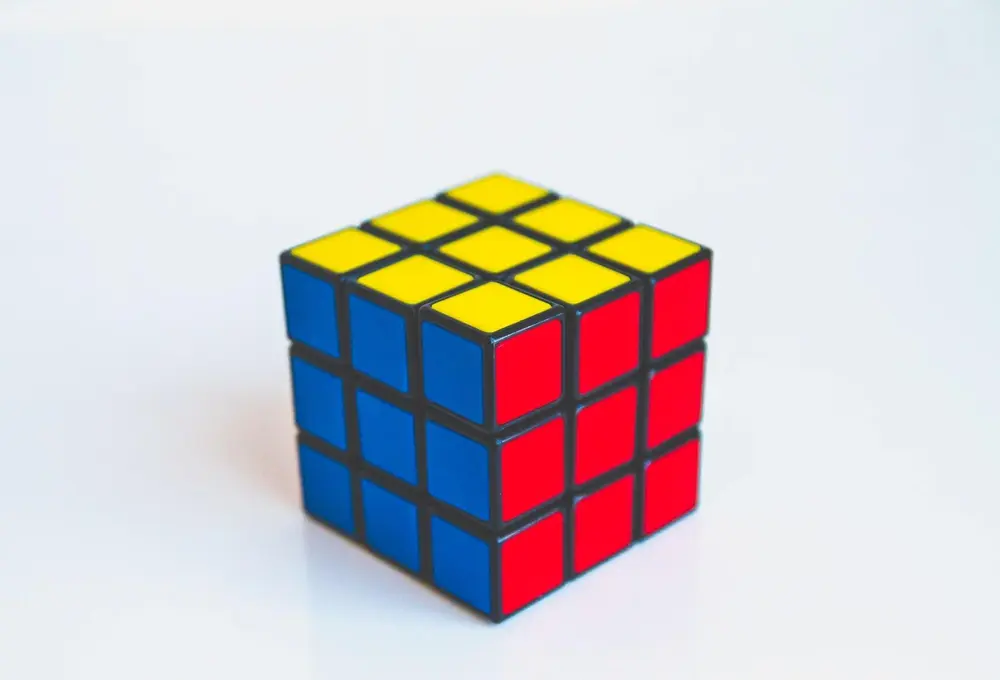# 3D shapes

For GCSE mathematics you need to be able to work with three dimensional objects. Normally, you have to work out problems such as volume and surface area. Also you have to know how to use three-dimensional co-ordinates.

Three dimensional co-ordinates are written in the form ( x , y , z ). The axis can be in any direction, however the labels on the axis always go around in order.

Here is an example:

• A - ( 0 , 2 , 2 )
• B - ( 3 , 2 , 2 )
• C - ( 3 , 2 , 0 )
• D - ( 3 , 0 , 0 )

With co-ordinates you can work out the equation between the two axes. This equation would represent a line. However, with three dimensional co-ordinates, the equation between two of the axes would give the equation to a plain.

Shape and Space

Shape and Space

Shape and Space

Shape and Space

Shape and Space

Shape and Space